# Why don't all materials behave like magnets?

## Magnetism¶

People have known and used magnetic phenomena since ancient times. In particular, the use of thin, rotatable magnetic disks in compasses was in many ways "trend-setting" for the development of mankind. In the meantime, electromagnets, electric motors, generators and transformers in particular have become an integral part of our modern society.

### Permanent magnets¶

Probably the best known is the attraction of magnets on iron. Substances that, like iron, are attracted by magnets and become magnetized themselves, are called "ferromagnetic" or colloquially as "magnetic" after the Latin name of iron ("ferrum"). These include cobalt, nickel, neodymium and a few alloys.

Magnets have (almost) no effect on non-ferromagnetic materials.

The model of elementary magnets

Magnets always have two opposing areas where their magnetic effect is particularly strong. These areas are called magnetic poles.

If a magnet breaks into two parts, you can see that both parts have two magnetic poles. Even with further division, the magnetic poles always appear in pairs. Conversely, each magnet can be understood from a large number of the smallest "elementary magnets":

• If all elementary magnets of a magnet point in the same direction in an orderly manner, their effect is increased - the result is a strong magnetic field.
• If the elementary magnets point in different directions, their effects cancel each other out.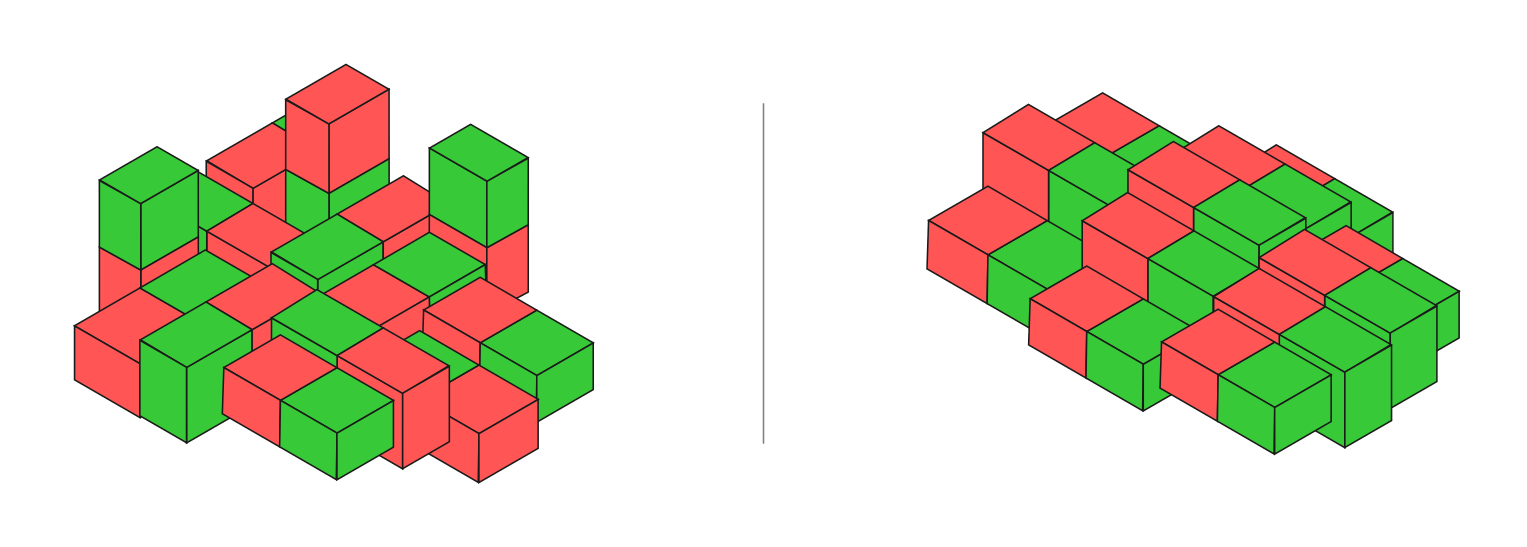The elementary magnets of a magnetizable material have different orientations. In a magnetized material, however, the elementary magnets have a uniform magnetic order.

In contrast to electrical charges, magnets do not have “mono-poles”, but only “di-poles”: In a magnet, the north and south poles never appear individually, but always in combination.

Magnetic Influence

In non-magnetized iron, the elementary magnets are arranged in areas. These so-called “Weiss districts”, however, have different orientations, so that a piece of iron does not exert any magnetic effect on other bodies.

Iron can become a magnet itself by approaching a magnet. During this process, known as "magnetic influence", the individual elementary magnets follow the external magnetic field like compass needles. Since the effects of the individual elementary magnets add up, the iron also acts as a magnet to the outside world.

The extent to which the magnetic order is retained when the external magnet is removed depends on the composition of the magnetized material:

• In the case of magnetically "soft" materials, for example soft iron, the magnetism caused by influence (largely) disappears again.
• In magnetically "hard" materials, such as steel, the magnetic effect is permanently retained.

Vibrations or strong heating can destroy the order of the elementary magnets again. A certain temperature (“Curie temperature”) can be specified for all substances, above which the thermal movement of the substance particles prevents a magnetic order. For example, iron is off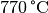no longer magnetizable.

### The magnetic field¶

The force that a magnet exerts on another magnetic body depends on the distance between the magnets, their shape and their orientation to one another.

A magnet shows the strongest force at its two opposite poles:

• Magnetizable bodies (e.g. iron paper clips) are equally strongly attracted by both magnetic poles (magnetic influence).
• When their magnetic poles approach, permanent magnets and electromagnets experience a strong attractive or repulsive effect, depending on their orientation. If the magnetic poles - based on the earth's magnetic field - are referred to as the north and south poles, it can be seen that north and south poles attract each other, while north and north poles or south and south poles repel each other.

The magnetic force is strongest in the immediate vicinity of the magnetic poles and decreases with increasing distance from the magnetic poles.

The easiest way to observe the dependence of the magnetic forces on the shape of the magnet is with the help of iron filings. These are deflected or attracted to different degrees at different points around the magnet, so that in each case a pattern that is characteristic of the shape of the magnet results.

When moved around a magnet, a magnetic needle also deflects itself at every point in the same direction as the iron filings, with the north pole of the magnetic needle always oriented towards the south pole of the magnet.

The closed lines that result in the patterns of the iron filings around the magnet thus indicate the direction of the magnetic force. This spatial distribution of the magnetic forces around the magnet is referred to as the "magnetic field" and is represented in technical drawings (somewhat simplified) by a series of closed lines. Often small arrows are drawn along these "magnetic field lines", each at the north pole from the Magnet out and re-enter at the South Pole.

In this way, both the force effect of a magnet can be visualized and its influence on other magnetic or magnetizable bodies can be estimated.

Summary:

• Magnetic poles always appear in pairs. Along these, the magnetic field lines run on continuously closed paths.
• The arrows of the magnetic field lines point inside the magnet in the direction of the north pole, outside the magnet in the direction of the south pole.
• The same magnetic poles repel each other, different magnetic poles attract each other.
• The magnetic effect decreases with increasing distance from the magnetic poles.
• If several magnets are aligned in the same direction, their effects increase to form an overall magnetic field. If the orientation changes, (almost) no magnetic effect can be detected towards the outside.
• Magnetic fields can already be shielded by thin magnetizable plates (made of iron, for example), but non-magnetizable materials penetrate almost without attenuation.

### Electromagnet¶

In 1820, Hans-Christian Oersted discovered that a current-carrying electrical conductor also exerts a (weak) force on a nearby compass needle. This statement contradicted the prevailing view that electricity and magnetism were two separate phenomena.

By positioning the electrical conductor and the magnetic needle (s) in different positions, Örsted was able to determine the weak magnetic field of the conductor in the same way as with permanent magnets. He could observe the following law:

Right-hand rule (for conductor tracks):

If you hold a straight conductor through which current flows with your right hand so that the outstretched thumb points along the technical direction of the current (from to), then the remaining fingers indicate the direction of the magnetic field lines.

The right-hand rule also applies when the electrical conductor is bent into a circular shape. The thumb must be carried along with the curvature of the conductor in order to always point in the technical direction of the current. It turns out that the resulting magnetic field always points in the same direction at the various points on the conductor loop.

Example:

• If the conductor loop is on a table and the technical current direction is counterclockwise, the magnetic field inside the conductor loop points vertically upwards at every point. (On the outside of the conductor loop, it points vertically downwards.)

The force effects at the individual points of the electrical conductor add up in a conductor loop to form an overall magnetic field that is reminiscent of the shape of a ring magnet. This effect can be significantly increased by laying several conductor loops on top of one another or by winding a long conductor into a coil.

The magnetic field of a current-carrying coil

A long current-carrying coil with many turns generates a magnetic field on the outside that is very similar to that of a bar magnet. The right-hand rule again provides information about the direction in which the north or south pole of the "electromagnet" is located.

Right-hand rule (for coils):

If you hold the turns of a coil with your right hand so that the fingers point in the technical direction of the current, then the magnetic north pole of the coil is in the direction of the outstretched thumb.

The magnetic effect of a current-carrying coil can be increased by placing a piece of non-magnetized iron through the coil. Due to the magnetic influence, the iron itself becomes magnetic and considerably increases the magnetic effect of the coil.

Electromagnets have the following advantages over permanent magnets:

• Electromagnets can be switched on and off.
• The strength of an electromagnet can be regulated by the strength of the current in the coil.
• The north and south poles of an electromagnet can be swapped by swapping the plus and minus poles of the applied voltage.
• Electromagnets are almost unbreakable, do not lose strength over time, and are inexpensive to manufacture.

### Magnetic field strength and magnetic flux density¶

The force effect of a magnetic field can be quantified by the so-called magnetic field strength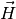to be discribed.

With a straight conductor track, the magnetic field strength is proportional to the flowing current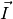and inversely proportional to the distance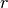from the conductor track. So the following applies: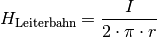The unit of the magnetic field strength is according to the above formula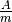.

Inside a conductor loop, the value of the magnetic field strength is a factor of that of a straight conductor path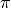increased, so it applies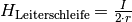. Combine a number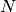on conductor loops to form a long coil, the magnetic field strength inside the coil is almost "homogeneous", so the field lines run parallel to each other. For the magnetic field strength of a coil with one number of turnsand a lengthapplies:

A second important parameter for describing magnetic fields is the so-called magnetic flux density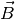. It clearly shows how many field lines go through a certain area. There is the following relationship between the magnetic flux density, which characterizes the spatial distribution of the field lines, and the magnetic field strength, which describes the force effect on other magnets:

Here referred to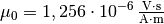the magnetic field constant. The unit for the magnetic flux density is: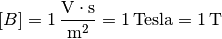For example, the magnetic flux density of the earth is around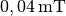.

Strictly speaking, the above relationship (2) only applies in a vacuum, but to a good approximation also in air. However, if there is another material in the magnetic field, its so-called permeability must be taken into account, which indicates whether the field lines running through the material are more likely to be contracted by the material or pushed out of the material. In general, the following applies: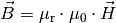For the size of the permeability number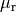there are essentially three different cases:

• In diamagnetic materials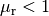, The magnetic flux density is therefore slightly reduced compared to the external field. Examples: copper, zinc, water, nitrogen.
• In paramagnetic materials is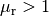, The magnetic flux density is thus slightly increased compared to the external field. Examples: aluminum, platinum, oxygen.
• In ferromagnetic materials it is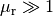, is the magnetic flux density is therefore greatly increased compared to the external field. Examples: iron, cobalt, nickel.

The permeability numbers of dia- and paramagnetic materials are usually only a littledifferent, so that the magnetic properties of these materials rarely play a role in technical applications.

### The Lorentz Force¶

While every flow of electrical current results in a magnetic field, every magnetic field has an influence on an electrical current.

If a piece of a current-carrying conductor is in a magnetic field, a force acts on the piece of conductor. It is called the "Lorentz force" after its discoverer Hendrik Antoon Lorentz. The amount of force depends on the strength of the current flow or magnetic field as well as the angle between the conductor and the magnetic field:

• The magnitude of the force is greatest when the conductor is oriented perpendicular to the magnetic field.
• If the conductor and the magnetic field point in the same direction, there is no force.

The Lorentz force always acts perpendicular to the direction of the current and perpendicular to the magnetic field. The following rule of thumb applies to the relationship between current flow, magnetic field and direction of the acting force:

Three-finger rule:

If the thumb of the right hand points in the direction of the technical current direction (from to) and the index finger in the direction of the magnetic field, the middle finger indicates the direction of the force acting.

One denotes the length of the stream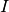traversed conductor piece, which is in the magnetic fieldis located with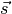so can the Lorentz force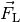can be calculated quantitatively using the following formula:

If the current-carrying conductor runs perpendicular to the magnetic field, the amount of the Lorentz force is the same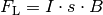, otherwise results in the vector product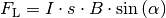, in which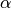indicates the angle between the conductor piece and the magnetic field. Not just a single piece of conductor, but also a coil, for example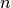If windings are introduced into the magnetic field, this also results in a-fold path length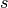and thus a-fold force effect; this is used, for example, in the construction of electric motors.

Movement of charged particles in magnetic fields

An electric current corresponds to a temporal transport of electric charge. If you put the context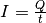between the amperage, the amount of cargo transported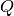and the time it takesinto which the above formula (3) follows: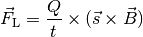This equation can also be written like this: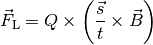The ratio of the distanceand timecan be called speed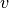to be written. Thus it results for one with a chargecharged particle moving with speed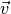by a magnetic field with a flux densityemotional:

In this case, too, the vector product gives a maximum value when the particle moves perpendicular to the magnetic field; otherwise it can be used for the vector product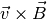also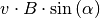be written, whereindicates the angle between the direction of movement of the charged particle and the direction of the magnetic field. The above equation can also be written like this: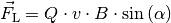Is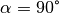, then applies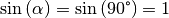, and the Lorentz force takes its maximum value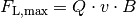at.

Application: mass spectrometer

The Lorentz force can be used to determine the so-called “specific charge” of a particle, that is, the ratio of its chargeand its mass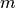to determine. To do this, the particle (or a beam of similar particles) is allowed to travel at one speedenter a magnetic field perpendicular to the magnetic field lines.

If a charged particle, for example an ion or an electron, moves in the horizontal plane and the magnetic field is vertical, the speed remainsof the particle even if it is deflected by the Lorentz force perpendicular to the magnetic field. If the arrangement is in a vacuum, there are no frictional forces, so the value of the speed isof the particle constant. If you also keep the magnetic flux density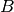of the magnetic field is constant, the amount of the Lorentz force also remains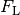constant.

The Lorentz force forces the charged particle to follow a circular path through continuous deflection. The necessary radial force must be used as a condition of equilibrium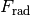and the Lorentz forcebe the same size: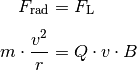For the specific charge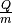of the particle we get: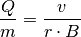Is the flux densityof the magnetic field as well as the entry velocityknown so can by measuring the radiusthe circular path can be closed to the specific charge of the particle; such an arrangement is called a mass spectrometer.

If the particle beam involves ions each with the same charge, a mass spectrometer can in particular determine the ratio in which individual isotopes of the ion occur; these have largely the same chemical and physical properties, but vary in their mass. In a mass spectrometer, isotopes with a larger mass are directed onto circular paths with a larger radius, so that the frequency distribution of the individual isotopes can be measured using appropriate detectors.

Application: Hall sensors

The Lorentz force is also used in "Hall sensors" named after Edwin Hall, which can be used to measure magnetic flux densities.

A Hall sensor basically has the following structure:

The electrons in a Hall sensor are deflected by the Lorentz force until an equally large, oppositely directed force is established by the electrical field that is building up. If this is the case, the following equilibrium prevails: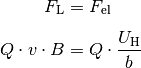The electrical voltage that is established in the case of equilibrium becomes "Hall voltage"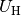called; it can be tapped and measured at corresponding connections along the broad side of the plate. According to the above equation, the following applies to the Hall voltage:

If one knows the speed of the electrons, which occurs depending on the semiconductor material when the current flows through the plate, then the magnetic flux density can be determined directly by measuring the Hall voltagean external magnetic field can be calculated.

Hall sensors have a wide variety of applications in practice, especially because they are very resistant to water, dirt and vibrations. They are used, for example, in so-called “current clamps” for non-contact measurement of currents in electrical conductors and in cable locators; Both cases take advantage of the fact that electrical currents in conductors cause a magnetic field that can be measured with Hall sensors. Hall sensors are also used for speed measurement in vehicles and turbines; Only small magnets have to be built into the objects rotating past the Hall sensor, which cause a short voltage signal with each rotation of the sensor, which can be registered with a suitable measuring circuit.

### Electromagnetic induction¶

If you move a piece of metal perpendicular to the field lines of a homogeneous magnetic field, the Lorentz force inside the conductor causes the electrons to be displaced perpendicular to the direction of movement and also perpendicular to the direction of the magnetic field. Since only the free electrons move, but not the positively charged atomic nuclei, an electric field or voltage builds up inside the metal between the two ends.

This process is called electromagnetic induction. If you connect a measuring device or a consumer (for example a small light bulb) to the ends of the conductor, the induced voltage can in principle be measured or used as a power source. With a single short section of conductor, however, only very low voltage values ​​in the milivolt range result; higher voltage or current values ​​are obtained if the individual conductor section is replaced by a coil with as many turns as possible.

The above figure also shows the technical direction of the current that results from the specified mechanical force when the ladder swing moves (Lorentz force or three-finger rule). If no consumer or measuring device is connected, an electric field builds up in the same direction due to the shifting of charges inside the ladder swing. In the case of equilibrium, the Lorentz force, which the electrons experience inside the conductor section moved by the magnetic field, is balanced by the resulting electric field: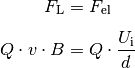The resulting voltage becomes induction voltage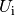called,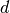describes the length of the conductor that is in the magnetic field. Will follow the above equationdissolved, it results: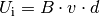Induction voltage only occurs when the conductor is moving at a speedis moved perpendicular to the magnetic field. If the speed has the value zero or if the movement runs parallel to the magnetic field lines, the induced voltage is zero.

### Faraday’s law of induction¶

A more general formula for describing the voltage occurring in electromagnetic inductionTo be able to set up, it makes sense to have a new size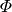to define what is called "magnetic flux":

Between the magnetic fluxand the magnetic flux density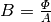there is a similar relationship to that between the current intensityand the current density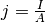: The latter quantities each indicate the areal density of the reference quantity. The magnetic flux density is not about charge carriers that migrate through a certain area, but about the number of field lines that pass through it. The magnetic flux describes the amount of field lines that pass through an area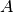run away.

Is the magnetic fluxThe induced voltage is constant over time through an areaequals zero. If, on the other hand, the magnetic flux changesby optionally choosing the strengththe magnetic flux density and / or the size of the area through which the magnetic field flowschanges, it becomes an induction voltagegenerated, which is the greater, the faster this change occurs. The relationship for the induction voltage in a conductor loop is:

This law, according to which the voltage induced in a conductor loop is equal to the change in the magnetic flux over time, was first discovered in 1831 by Michael Faraday.

Examples:

• In a homogeneous and temporally constant magnetic field, there can be a change in the area through which the magnetic field flowsbe effected on the one hand by moving the conductor loop into or out of the magnetic field; on the other hand, the same effect, as can be seen in the following figure, can also be brought about by rotating the conductor loop in the magnetic field.

• If you move a bar magnet through a stationary conductor loop, the magnetic flux density of which is not homogeneous (but increases towards the poles), the conductor loop feels a magnetic field that changes over time. Here, too, a voltage (albeit a very low one) is induced.

The two possibilities described above for producing an induction voltage can be optimized by using a coil consisting of as many turns as possible instead of a single conductor loop. For the induction voltage of a coil withTurns:

Lenz's rule

In the above equations (7) and (8) for describing the induction voltage, the change over time is in front of the time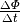a minus sign. This is a consequence of a law discovered by Emil Lenz, according to which the induction voltage always counteracts its cause.

The cause of the induction voltage is ultimately the Lorentz force, which causes a shift of the electrons and thus an electric field inside the moving conductor loop (s). However, this field does not accelerate the electrons any further (which would result in a perpetual motion machine), but rather slow them down.

... to be continued ...